Chi Tran

•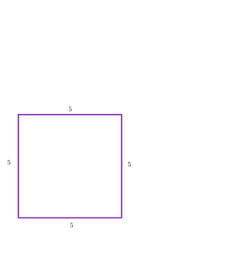45-45-90 Triangle

Activity

Chi Tran

•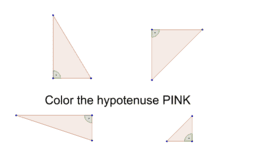Find the Hypotenuse

Activity

Chi Tran

•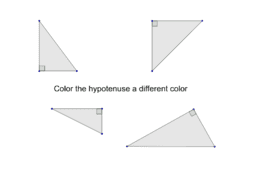Find the Hypotenuse (6 December 2015)

Activity

Chi Tran

•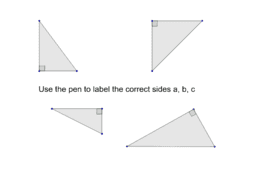Pythagorean Theorem (28 January 2016)

Activity

Chi Tran

•Artwork Dilation

Activity

Chi Tran

•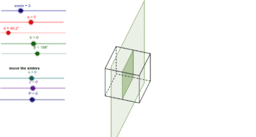Cross Sections of a Rectangular Prism

Activity

Chi Tran

•Cross Sections of a Rectangular Pyramid

Activity

Chi Tran

•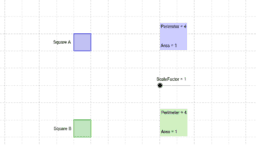Perimeter Scale Factor vs Area Scale Factor

Activity

Chi Tran

•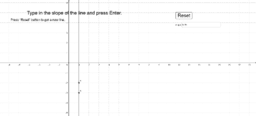Finding the slope of a line

Activity

Chi Tran

•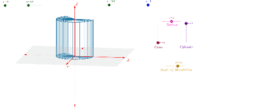Cone & Cylinder by Revolution

Activity

Chi Tran

•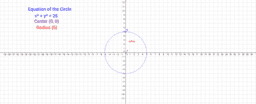Investigating the Equation of a Circle

Activity

Chi Tran

•Investigating Reflection over the Y-Axis

Activity

Chi Tran

•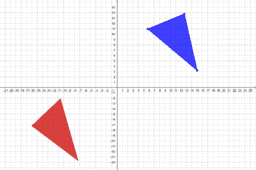Congruence by Rigid Motions

Activity

Chi Tran

•Geometry

Book

Chi Tran

•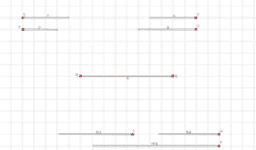Triangle Inequality

Activity

Chi Tran

•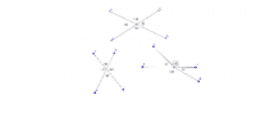Vertical Angles

Activity

Chi Tran

•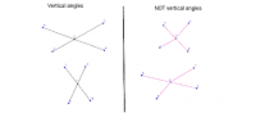Vertical Angles

Activity

Chi Tran

•Complementary Supplementary Angles

Activity

Chi Tran

•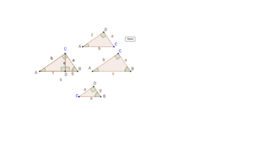Similar Right Triangles

Activity

Chi Tran

•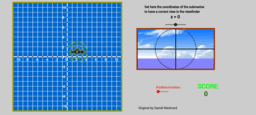Coordinate Activity : Submarine

Activity

Chi Tran

•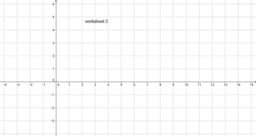worksheet 2

Activity

Chi Tran

•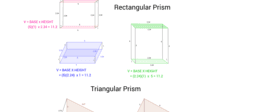Prisms

Activity

Chi Tran

•Trig as Ratios

Activity

Chi Tran

•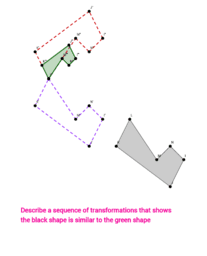Activity

Chi Tran